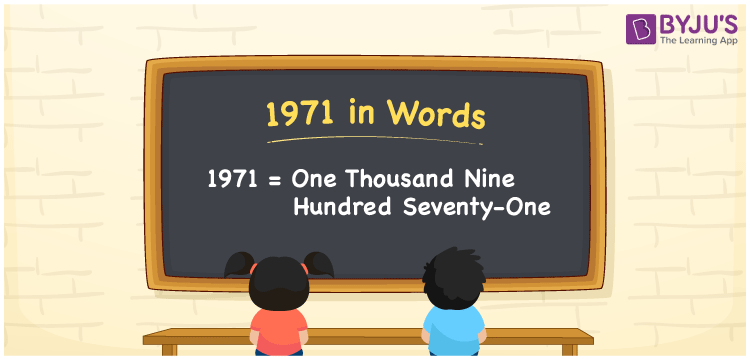# 1971 in Words

We can write 1971 in words as One thousand nine hundred seventy-one. If you bought a bean bag for Rs. 1971, then you can say, “I bought a bean bag for One thousand nine hundred seventy-one rupees”. Here, One thousand nine hundred seventy-one represents the number 1971, and this is called the number name of 1971. Also, we know that 1971 is a cardinal number.

 1971 in words One thousand nine hundred seventy-one One thousand nine hundred seventy-one in Numbers 1971

## 1971 in English Words

Generally, we write numbers in words using the English alphabet. Thus, we spell 1971 in English as “One thousand nine hundred seventy-one”.## How to Write 1971 in Words?

Let us create a place value chart for 1971 to get its word form. The below table shows place values for all the four digits of 1971.

 Thousands Hundreds Tens Ones 1 9 7 1

Here, ones = 1, tens = 7, hundreds = 9, and thousands = 1.

The above numbers can be expanded as follows.

1 × Thousand + 9 × Hundred + 7 × Ten + 1 × One

= 1 × 1000 + 9 × 100 + 7 × 10 + 1 × 1

= 1000 + 900 + 70 + 1

= One thousand + Nine hundred + Seventy + One

= One thousand nine hundred seventy-one

Therefore, 1971 in words = One thousand nine hundred seventy-one.

1971 is a natural number that precedes 1972 and succeeds 1970.

1971 in words – One thousand nine hundred seventy-one

Is 1971 an even number? – No

Is 1971 an odd number? – Yes

Is 1971 a prime number? – No

Is 1971 a composite number? – Yes

Is 1971 a perfect square number? – No

Is 1971 a perfect cube number? – No

## Frequently Asked Questions on 1971 in Words

Q1

### How do you write 1971 in words?

We can write 1971 in words as One thousand nine hundred seventy-one.
Q2

### How to write Rs. 1971 in words on a cheque?

On a cheque, we generally write Rs. 1971 in words as “One thousand nine hundred seventy-one rupees only”.
Q3

### Write 1971.12 in words.

The decimal number 1971.12 can be written in words as “One thousand nine hundred seventy-one point one-two”.